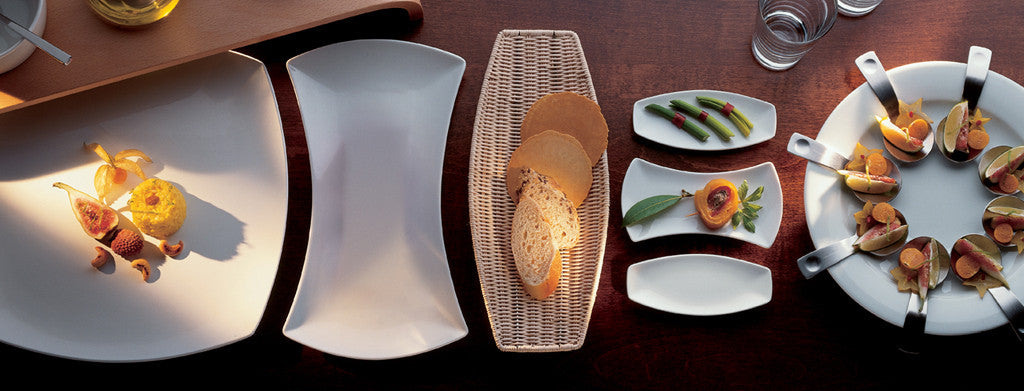Shopping Cart

### Refine

View all

#### Range# Crockery£4.04 each (ex VAT)£0.93 each (ex VAT)£1.02 each (ex VAT)£3.75 each (ex VAT)£3.61 each (ex VAT)£3.85 each (ex VAT)£1.97 each (ex VAT)£1.80 each (ex VAT)£3.57 each (ex VAT)£2.07 each (ex VAT)£3.50 each (ex VAT)£2.07 each (ex VAT)£4.02 each (ex VAT)£3.50 each (ex VAT)£4.04 each (ex VAT)£4.04 each (ex VAT)£3.75 each (ex VAT)£3.55 each (ex VAT)£3.50 each (ex VAT)£3.53 each (ex VAT)£24.94 each (ex VAT)£22.69 each (ex VAT)£23.57 each (ex VAT)£1.70 each (ex VAT)£3.83 each (ex VAT)£1.70 each (ex VAT)£1.38 each (ex VAT)£1.94 each (ex VAT)£0.83 each (ex VAT)£3.69 each (ex VAT)£2.07 each (ex VAT)£1.29 each (ex VAT)£1.12 each (ex VAT)£3.38 each (ex VAT)£1.31 each (ex VAT)£1.91 each (ex VAT)£3.99 each (ex VAT)£3.99 each (ex VAT)£3.99 each (ex VAT)£3.99 each (ex VAT)£3.99 each (ex VAT)£3.99 each (ex VAT)£3.99 each (ex VAT)£3.99 each (ex VAT)£3.49 each (ex VAT)£3.49 each (ex VAT)£3.99 each (ex VAT)£3.99 each (ex VAT)